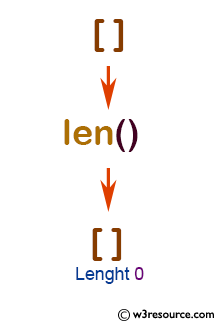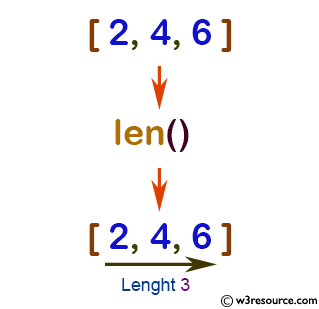# Python: len() function

## len() function

The len() function is used to get the length of an object.

Version:

(Python 3.2.5)

Syntax:

```len(s)
```

Parameter:

Name Description Required /
Optional
s A sequence (string, bytes, tuple, list, or range) or a collection (dictionary, set or frozen set). Required

Return value:

The number of items of an object.

Example: Python len() function

``````ExampleList = []
print(ExampleList, 'length is', len(ExampleList))
``````

Output:

```[] length is 0
```

Pictorial Presentation:Example: Python len() function with List

``````ExampleList = [2, 4, 6]
print(ExampleList, 'length is', len(ExampleList))
``````

Output:

```[2, 4, 6] length is 3
```

Pictorial Presentation:Example: Python len() with Tuple

``````
ExampleTuple = (2, 4, 6)
print(ExampleTuple, 'length is', len(ExampleTuple))
``````

Output:

```(2, 4, 6) length is 3
```

Example: Python len() with Range

``````
ExampleRange = range(3, 12)
print('Length of', ExampleRange, 'is', len(ExampleRange))
``````

Output:

```Length of range(3, 12) is 9
```

Python Code Editor:

Previous: iter()
Next: list()

Test your Python skills with w3resource's quiz

﻿

## Python: Tips of the Day

How do I check if a list is empty?

For example, if passed the following:

```a = []
if not a:
print("List is empty")
```

Ref: https://bit.ly/2A4JXx9